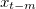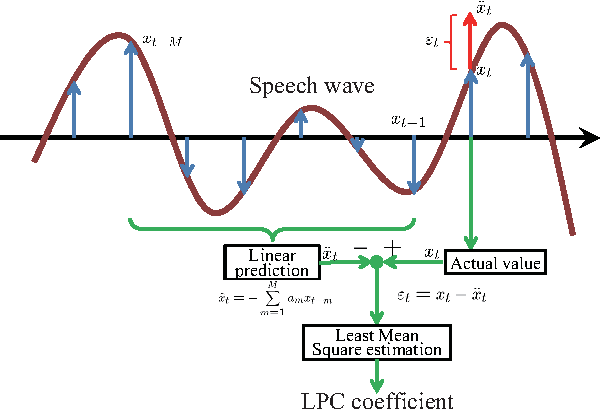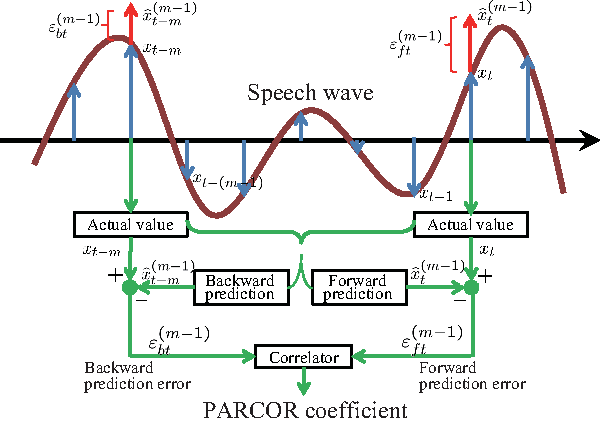# 10.1 Introduction

Problem

Read this section to learn about the features available for speech recognition.

Solution

The features used for common speech recognition include:

1. LPC (Linear Predictive Coding: Linear prediction) coefficient

2. PARCOR (PARcial CORelated: Partial autocorrelation) coefficient

3. MFCC (Mel-Frequency Cepstrum Coefficient)

4. MSLS (Mel-Scale Log Spectrum)

HARK supports only MFCC and MSLS. For speech recognition using an acoustic model distributed on the web, use MFCC. For speech recognition based on missing feature theory, MSLS is better than MFCC.

Discussion

The LPC coefficient is a parameter of a model of a spectrum envelope. It is based on the value at time of $t$ in the stationary process $x_ t$ being correlated with that of a recent sample. Figure 10.1 shows how to obtain the LPC coefficient. The LPC coefficient is a prediction coefficient ($a_ m$), in which the mean square error of the value ($\hat x_ t$) predicted from that of $M$ input signals in the past and the value $x_ t$ of actual input signals are minimal. Since this LPC yields a comparatively precise speech model, it has been used widely for speech analysis-synthesis. However, a model based on LPC has a high coefficient of sensitivity and may become unstable due to a slight error in this coefficient. Therefore, speech analysis-synthesis is performed in the form of PARCOR.

PARCOR is a correlation coefficient of prediction errors of $x_ t$ (forward) predicted from $x_{t-(m-1)}, \ldots , x_{t-1}$ and $x_{t-m}$ (backward). Figure 10.2 shows how to derive this PARCOR. In principle, a model based on this PARCOR is stable  .

MFCC is a cepstrum parameter and is derived with filter banks placed at even intervals on a Mel frequency axis . Figure 10.3 shows its process of derivation.

MSLS is derived by a filter bank analysis similar to that of MFCC, without performing the reverse discrete cosine transformation, the final step of MFCC extraction processing, and is a feature remaining in the frequency domain. When the noise at a specific frequency is mixed with acoustic signals, specific features including the frequency are affected in MSLS. For MFCC, however, the influence of noise spreads and many features are affected. Therefore, in general, MSLS performs well when combining the missing feature theory for speech recognition.

 Hijiri Imai, sound signal processing, Morikita Shuppan Co., Ltd., 1996.

 Kiyohiro Shikano et al., IT Text speech recognition system, Ohmsha Co., Ltd., 2001.Figure 10.1: LPC coefficientsFigure 10.2: PARCOR coefficientsFigure 10.3: MFCC and MSLS